# November 2020 - Los Angeles, CA

ACADEMIC CREDENTIALS EVALUATION INSTITUTE, INC. (ACEI)
Founded in 1994, ACEI is a full-service company providing complete and integrated services in-house in the areas of international education research, credential evaluation, and translation. And, through our Global Consulting Group®, we offer our expertise in the following specialties: Media and Branding, Global Pathways, and Diversity, Equity and Inclusion (DEI) to interested institutions and organizations around the globe.

 table div table+table+table div table{width:100%;padding:0}table div table+table+table div table img{width:96.23%;padding:0;float:none}table div table+table+table div table td{width:100%;padding:0 1.88% 18px}/* styles */table div table+table+table+table+table div table{width:100%;padding:0}table div table+table+table+table+table div table img{width:96.23%;padding:0;float:none}table div table+table+table+table+table div table td{width:100%;padding:0 1.88% 18px}/* styles */table div table+table+table+table+table+table+table+table div table{width:100%;padding:0}table div table+table+table+table+table+table+table+table div table img{width:96.23%;padding:0;float:none}table div table+table+table+table+table+table+table+table div table td{width:100%;padding:0 1.88% 18px}/* styles */# Education News From Around the GlobePhoto Source: DocumentaryTube.com

# Syrian Refugees Switching Academic Paths

Five years after more than half a million Syrians arrived in Germany as refugees, many are taking different academic and career paths from the ones they had chosen in Syria. To read more, click here.

 table div table+table+table+table+table+table+table+table+table+table+table+table div table{width:100%;padding:0}table div table+table+table+table+table+table+table+table+table+table+table+table div table img{width:96.23%;padding:0;float:none}table div table+table+table+table+table+table+table+table+table+table+table+table div table td{width:100%;padding:0 1.88% 18px}/* styles */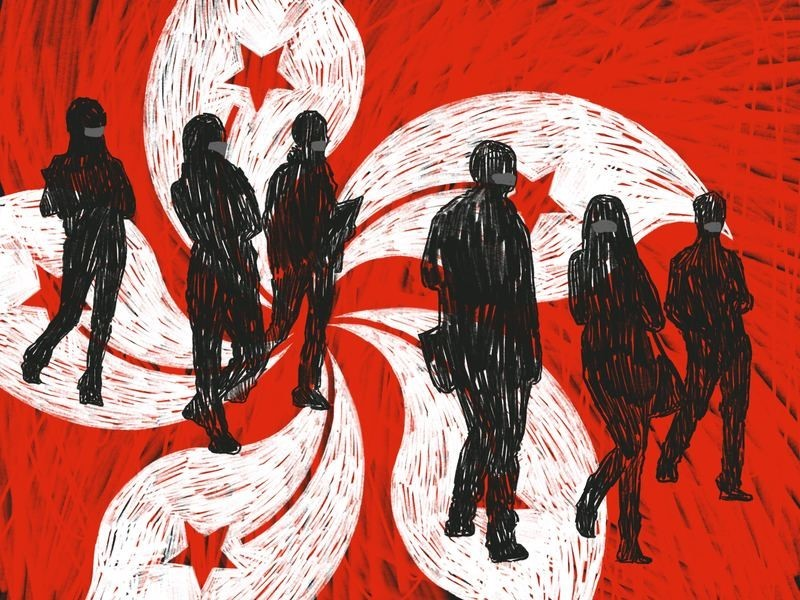Photo Credit: Sara Allam; Source: The Daily Orange

## HONG KONG

Student union chair Edy Jeh, who won a seat on HKU’s governing Council as an undergraduate representative earlier this month, fears several planned changes may erode academic freedom at Hong Kong’s oldest university. To read more, click here.

 table div table+table+table+table+table+table+table+table+table+table+table+table+table+table+table div table{width:100%;padding:0}table div table+table+table+table+table+table+table+table+table+table+table+table+table+table+table div table img{width:96.23%;padding:0;float:none}table div table+table+table+table+table+table+table+table+table+table+table+table+table+table+table div table td{width:100%;padding:0 1.88% 18px}/* styles */# Plans to build 7,000 School to fill the Education Gap

The Iraqi government has announced that it plans to build 7,000 schools as part of its efforts to fill the shortage of educational institutes in the country. To read more, click here.

 table div table+table+table+table+table+table+table+table+table+table+table+table+table+table+table+table+table div table{width:100%;padding:0}table div table+table+table+table+table+table+table+table+table+table+table+table+table+table+table+table+table div table img{width:96.23%;padding:0;float:none}table div table+table+table+table+table+table+table+table+table+table+table+table+table+table+table+table+table div table td{width:100%;padding:0 1.88% 18px}/* styles */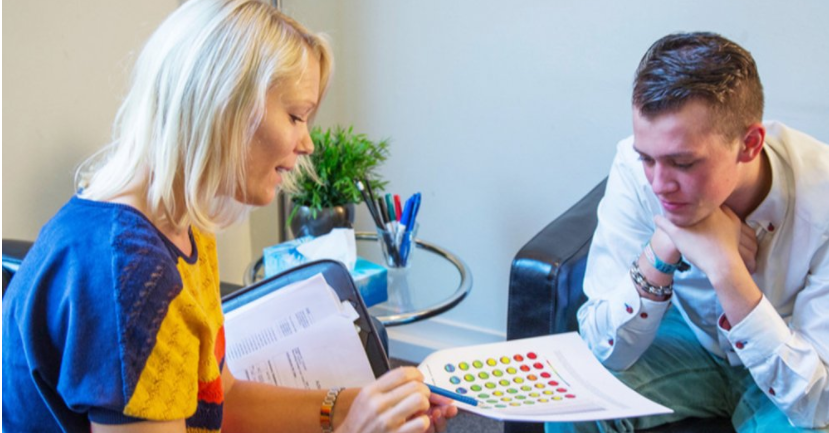Photo Source: Dutch Expat News

# Students Struggle with Mental Health

Educational institutions have been struggling to support students the way they had promised. Dutch student union LSVb is expressing concern for students in higher education, especially first-year students, who report feelings of loneliness, disproportionately high levels of stress and depression. To read more, click here.

 table div table+table+table+table+table+table+table+table+table+table+table+table+table+table+table+table+table+table+table+table div table{width:100%;padding:0}table div table+table+table+table+table+table+table+table+table+table+table+table+table+table+table+table+table+table+table+table div table img{width:96.23%;padding:0;float:none}table div table+table+table+table+table+table+table+table+table+table+table+table+table+table+table+table+table+table+table+table div table td{width:100%;padding:0 1.88% 18px}/* styles */# Democracy Group’s Call to Save Public Universities from Collapse

The Network for Democracy and Development (NDD) has called on Nigerian President Muhammadu Buhari and state governors to save public universities from avoidable collapse. This comes as public universities have remained shut for eight months owing to a strike by the Academic Staff Union of Universities (ASUU). To read more, click here.

 table div table+table+table+table+table+table+table+table+table+table+table+table+table+table+table+table+table+table+table+table+table+table div table{width:100%;padding:0}table div table+table+table+table+table+table+table+table+table+table+table+table+table+table+table+table+table+table+table+table+table+table div table img{width:96.23%;padding:0;float:none}table div table+table+table+table+table+table+table+table+table+table+table+table+table+table+table+table+table+table+table+table+table+table div table td{width:100%;padding:0 1.88% 18px}/* styles */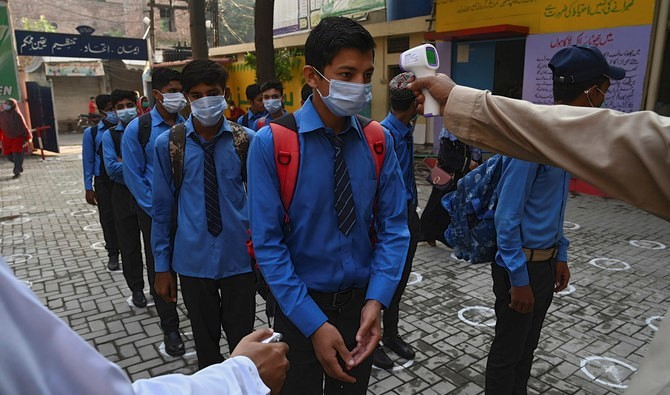Photo Credit: AFP; Source: Arab News

# Closure of Academic Institutions Starting November 26th

All educational institutions will be closed from November 26 till January 10 due to the rising number of coronavirus cases. To read more, click here.

 table div table+table+table+table+table+table+table+table+table+table+table+table+table+table+table+table+table+table+table+table+table+table+table+table+table div table{width:100%;padding:0}table div table+table+table+table+table+table+table+table+table+table+table+table+table+table+table+table+table+table+table+table+table+table+table+table+table div table img{width:96.23%;padding:0;float:none}table div table+table+table+table+table+table+table+table+table+table+table+table+table+table+table+table+table+table+table+table+table+table+table+table+table div table td{width:100%;padding:0 1.88% 18px}/* styles */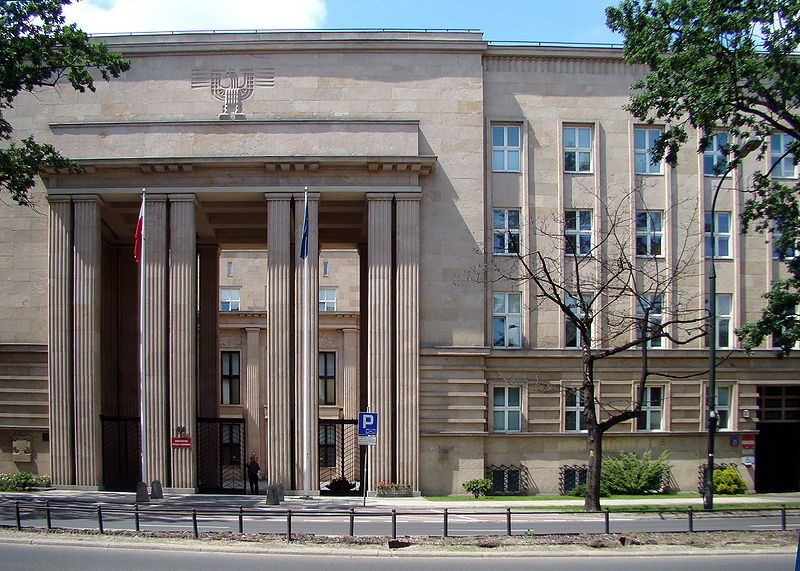Photo Source: WikimediaCommons

# Minister of Education Promises Legislation that Guarantees Freedom of Speech

Poland’s Minister of Education Przemyslaw Czarnek has announced that he will introduce legislative changes to guarantee “freedom of science” at universities, which, according to the minister, does not exist today. To read more, click here.

 table div table+table+table+table+table+table+table+table+table+table+table+table+table+table+table+table+table+table+table+table+table+table+table+table+table+table+table+table div table{width:100%;padding:0}table div table+table+table+table+table+table+table+table+table+table+table+table+table+table+table+table+table+table+table+table+table+table+table+table+table+table+table+table div table img{width:96.23%;padding:0;float:none}table div table+table+table+table+table+table+table+table+table+table+table+table+table+table+table+table+table+table+table+table+table+table+table+table+table+table+table+table div table td{width:100%;padding:0 1.88% 18px}/* styles */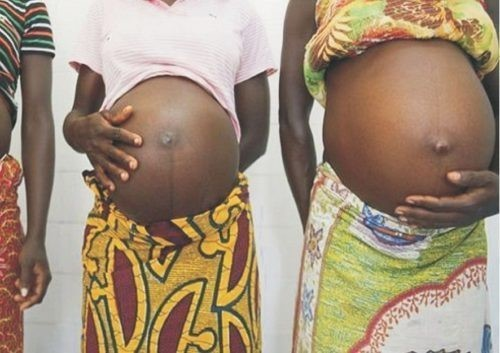Photo source: Sierra Leone Telegraph

# Court Case Filed to Overturn Ban Against Pregnant Girls from Attending School

International women’s rights organization – Equality Now, alongside its partner in Tanzania, last Friday filed a joint case at the African Court on Human and Peoples’ Rights against the Government of Tanzania, seeking to overturn the country’s discriminatory policy of permanently expelling pregnant girls from school and banning adolescent mothers from returning to school after giving birth. To read more, click here.

 table div table+table+table+table+table+table+table+table+table+table+table+table+table+table+table+table+table+table+table+table+table+table+table+table+table+table+table+table+table+table+table div table{width:100%;padding:0}table div table+table+table+table+table+table+table+table+table+table+table+table+table+table+table+table+table+table+table+table+table+table+table+table+table+table+table+table+table+table+table div table img{width:96.23%;padding:0;float:none}table div table+table+table+table+table+table+table+table+table+table+table+table+table+table+table+table+table+table+table+table+table+table+table+table+table+table+table+table+table+table+table div table td{width:100%;padding:0 1.88% 18px}/* styles */# Distance Learning is Better than No Education

It has been six months since the Education Directorate in the opposition-held province of Idlib launched a distance education project after schools’ repeated closure due to COVID-19 restrictions. This project is still operational even though schools were re-opened at the end of last September due to the continuing need and demand for remote-learning. To read more, click here.

 table div table+table+table+table+table+table+table+table+table+table+table+table+table+table+table+table+table+table+table+table+table+table+table+table+table+table+table+table+table+table+table+table+table div table{width:100%;padding:0}table div table+table+table+table+table+table+table+table+table+table+table+table+table+table+table+table+table+table+table+table+table+table+table+table+table+table+table+table+table+table+table+table+table div table img{width:96.23%;padding:0;float:none}table div table+table+table+table+table+table+table+table+table+table+table+table+table+table+table+table+table+table+table+table+table+table+table+table+table+table+table+table+table+table+table+table+table div table td{width:100%;padding:0 1.88% 18px}/* styles */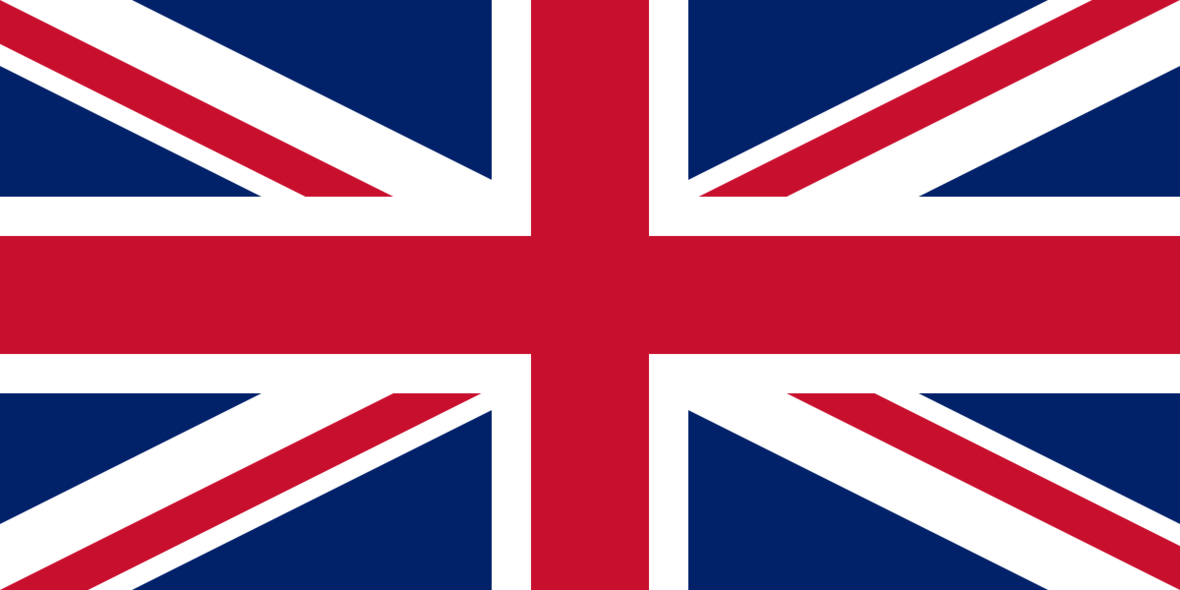# Watchdog threatens Sanctions if Universities let Quality Slip

Universities in the United Kingdom could face robust action if they allow quality to slip in certain degree subjects, or if they lower their expectations for students from disadvantaged backgrounds. To read more, click here.

 table div table+table+table+table+table+table+table+table+table+table+table+table+table+table+table+table+table+table+table+table+table+table+table+table+table+table+table+table+table+table+table+table+table+table+table div table{width:100%;padding:0}table div table+table+table+table+table+table+table+table+table+table+table+table+table+table+table+table+table+table+table+table+table+table+table+table+table+table+table+table+table+table+table+table+table+table+table div table img{width:96.23%;padding:0;float:none}table div table+table+table+table+table+table+table+table+table+table+table+table+table+table+table+table+table+table+table+table+table+table+table+table+table+table+table+table+table+table+table+table+table+table+table div table td{width:100%;padding:0 1.88% 18px}/* styles */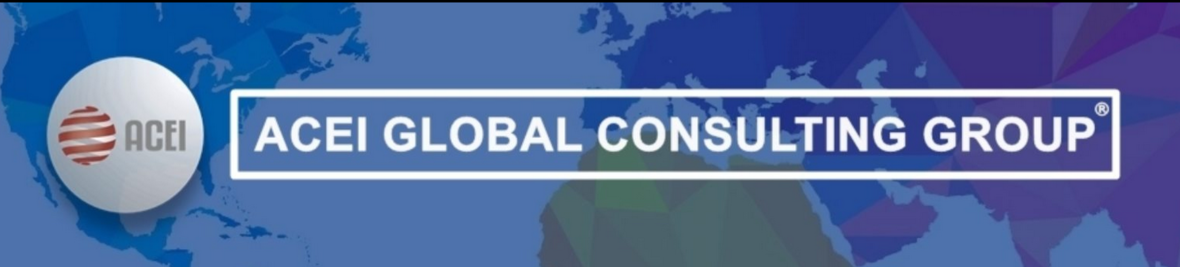table div table+table+table+table+table+table+table+table+table+table+table+table+table+table+table+table+table+table+table+table+table+table+table+table+table+table+table+table+table+table+table+table+table+table+table+table+table+table+table div table{width:100%;padding:0}table div table+table+table+table+table+table+table+table+table+table+table+table+table+table+table+table+table+table+table+table+table+table+table+table+table+table+table+table+table+table+table+table+table+table+table+table+table+table+table div table img{width:96.23%;padding:0;float:none}table div table+table+table+table+table+table+table+table+table+table+table+table+table+table+table+table+table+table+table+table+table+table+table+table+table+table+table+table+table+table+table+table+table+table+table+table+table+table+table div table td{width:100%;padding:0 1.88% 18px}/* styles */